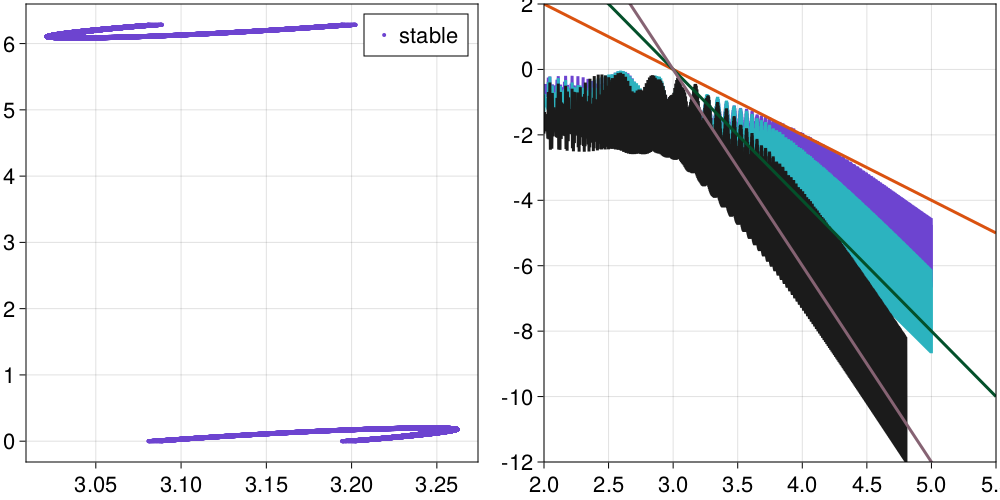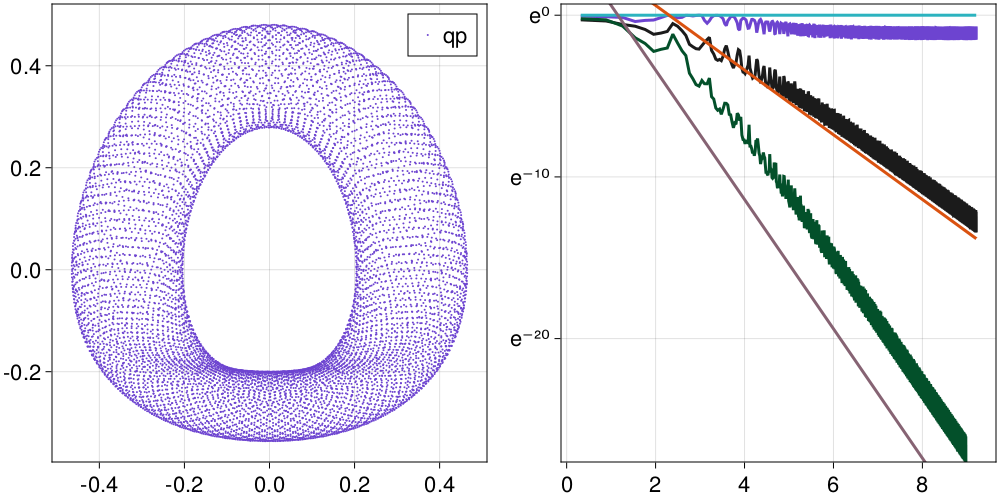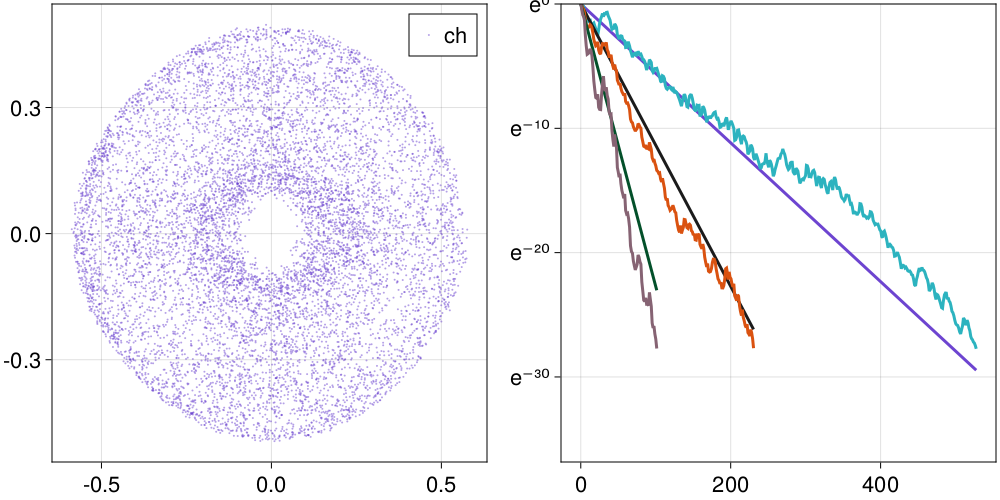# Detecting & Categorizing Chaos

Being able to detect and distinguish chaotic from regular behavior is crucial in the study of dynamical systems. Most of the time a positive maximum lyapunov exponent and a bounded system indicate chaos.

However, the convergence of the Lyapunov exponent can be slow, or even misleading, as the types of chaotic behavior vary with respect to their predictability. There are many alternatives, some more efficient and some more accurate in characterizing chaotic and regular motion. Some of these methods are included in DynamicalSystems.jl.

Performance depends on the solver

Notice that the performance of functions that use ContinuousDynamicalSystems depend crucially on the chosen solver. Please see the documentation page on Choosing a solver for an in-depth discussion.

## Generalized Alignment Index

"GALI" for sort, is a method that relies on the fact that initially orthogonal deviation vectors tend to align towards the direction of the maximum Lyapunov exponent for chaotic motion. It is one of the most recent and cheapest methods for distinguishing chaotic and regular behavior, introduced first in 2007 by Skokos, Bountis & Antonopoulos.

ChaosTools.galiFunction
gali(ds::DynamicalSystem, tmax, k::Int | Q0; kwargs...) -> GALI_k, t

Compute $\text{GALI}_k$[Skokos2007] for a given k up to time tmax. Return $\text{GALI}_k(t)$ and time vector $t$.

The third argument, which sets the order of gali, can be an integer k, or a matrix with its columns being the deviation vectors (then k = size(Q0)). In the first case random orthonormal vectors are chosen.

Keyword Arguments

• threshold = 1e-12 : If GALI_k falls below the threshold iteration is terminated.
• Δt = 1 : Time-step between deviation vector normalizations. For continuous systems this is approximate.
• u0 : Initial state for the system. Defaults to get_state(ds).
• diffeq is a NamedTuple (or Dict) of keyword arguments propagated into init of DifferentialEquations.jl. See trajectory for examples. Only valid for continuous systems.

Description

The Generalized Alignment Index, $\text{GALI}_k$, is an efficient (and very fast) indicator of chaotic or regular behavior type in $D$-dimensional Hamiltonian systems ($D$ is number of variables). The asymptotic behavior of $\text{GALI}_k(t)$ depends critically on the type of orbit resulting from the initial condition. If it is a chaotic orbit, then

$$$\text{GALI}_k(t) \sim \exp\left[\sum_{j=1}^k (\lambda_1 - \lambda_j)t \right]$$$

with $\lambda_j$ being the j-th Lyapunov exponent (see lyapunov, lyapunovspectrum). If on the other hand the orbit is regular, corresponding to movement in $d$-dimensional torus with $1 \le d \le D/2$ then it holds

$$$\text{GALI}_k(t) \sim \begin{cases} \text{const.}, & \text{if} \;\; 2 \le k \le d \; \; \text{and} \; \;d > 1 \\ t^{-(k - d)}, & \text{if} \;\; d < k \le D - d \\ t^{-(2k - D)}, & \text{if} \;\; D - d < k \le D \end{cases}$$$

Traditionally, if $\text{GALI}_k(t)$ does not become less than the threshold until tmax the given orbit is said to be chaotic, otherwise it is regular.

Our implementation is not based on the original paper, but rather in the method described in[Skokos2016b], which uses the product of the singular values of $A$, a matrix that has as columns the deviation vectors.

Performance Notes

This function uses a tangent_integrator. For loops over initial conditions and/or parameter values one should use the low level method that accepts an integrator, and reinit! it to new initial conditions. See the "advanced documentation" for info on the integrator object. The low level method is

ChaosTools.gali(tinteg, tmax, Δt, threshold)

(section 5.3.1 and ref.  therein), Lecture Notes in Physics 915, Springer (2016)

### Discrete Example

We will use 3 coupled standard maps as an example for a discrete system:

using DynamicalSystems
M = 3; ks = 3ones(M); Γ = 0.1;
stable = [π, π, π, 0.01, 0, 0] .+ 0.1
chaotic = rand(2M)

ds = Systems.coupledstandardmaps(M, stable; ks, Γ)
6-dimensional discrete dynamical system
state:       [3.24159, 3.24159, 3.24159, 0.11, 0.1, 0.1]
rule f:      CoupledStandardMaps
in-place?    true
jacobian:    CoupledStandardMaps
parameters:  [3.0, 3.0, 3.0, 0.1]

For this example we will see the behavior of GALI for a stable orbit

using CairoMakie
tr = trajectory(ds, 100000)

fig, ax = scatter(tr[:,1], tr[:,1+M]; label="stable", markersize=4)
axislegend(ax)

ax = Axis(fig[1,2])
for k in [4, 5, 6]
g, t = gali(ds, 1e5, k; threshold=1e-12)
lines!(ax, log10.(t), log10.(g); label="GALI_$(k)") end lt = 2:0.5:5.5 lines!(ax, lt, -2(lt .- 3), label="slope -2") lines!(ax, lt, -4(lt .- 3), label="slope -4") lines!(ax, lt, -6(lt .- 3), label="slope -6") xlims!(ax, 2, 5.5) ylims!(ax, -12, 2) fig### Continuous Example As an example of a continuous system, let's see the Henon-Heiles: using DynamicalSystems using CairoMakie, OrdinaryDiffEq Δt = 1.0 diffeq = (abstol=1e-9, retol=1e-9, alg = Vern9(), maxiters = typemax(Int)) sp = [0, .295456, .407308431, 0] # stable periodic orbit: 1D torus qp = [0, .483000, .278980390, 0] # quasiperiodic orbit: 2D torus ch = [0, -0.25, 0.42081, 0] # chaotic orbit ds = Systems.henonheiles(sp) 4-dimensional continuous dynamical system state: [0.0, 0.295456, 0.407308, 0.0] rule f: hhrule! in-place? true jacobian: hhjacob! parameters: nothing Let's see what happens with a quasi-periodic orbit: tr = trajectory(ds, 10000.0, qp; Δt, diffeq) fig, ax = scatter(tr[:,1], tr[:,3]; label="qp", markersize=2) axislegend(ax) ax = Axis(fig[1,2]; yscale = log) for k in [2,3,4] g, t = gali(ds, 10000.0, k; u0 = qp, Δt, diffeq) logt = log.(t) lines!(ax, logt, g; label="GALI_$(k)")
if k == 2
lines!(ax, logt, 1 ./ t.^(2k-4); label="slope -$(2k-4)") else lines!(ax, logt, 100 ./ t.^(2k-4); label="slope -$(2k-4)")
end
end
ylims!(ax, 1e-12, 2)
figFinally, here is GALI of a continuous system with a chaotic orbit

tr = trajectory(ds, 10000.0, ch; Δt, diffeq)
fig, ax = scatter(tr[:,1], tr[:,3]; label="ch", markersize=2, color = (Main.COLORS, 0.5))
axislegend(ax)

ax = Axis(fig[1,2]; yscale = log)
ls = lyapunovspectrum(ds, 5000.0; Δt, u0 = ch, diffeq)
for k in [2,3,4]
ex = sum(ls - ls[j] for j in 2:k)
g, t = gali(ds, 1000, k; u0 = ch, Δt = Δt, diffeq)
lines!(t, exp.(-ex.*t); label="exp. k=$k") lines!(t, g; label="GALI_$(k)")
end
ylims!(ax, 1e-16, 1)
figAs you can see, the results of both discrete and continuous systems match very well the theory described in gali.

### Using GALI

No-one in their right mind would try to fit power-laws in order to distinguish between chaotic and regular behavior, like the above examples. These were just proofs that the method works as expected in all cases.

The most common usage of $\text{GALI}_k$ is to define a (sufficiently) small amount of time and a (sufficiently) small threshold and see whether $\text{GALI}_k$ stays below it, for a (sufficiently) big $k$.

using DynamicalSystems, PyPlot

function main(k)
# Measure of chaoticity: final time of gali_2
dens = 201
chaoticity = zeros(Int, dens, dens)

θs = ps = range(0, stop = 2π, length = dens+1)
ds = Systems.standardmap(k = k)

tinteg = tangent_integrator(ds, 2)

for (i, θ) ∈ enumerate(θs[1:dens])